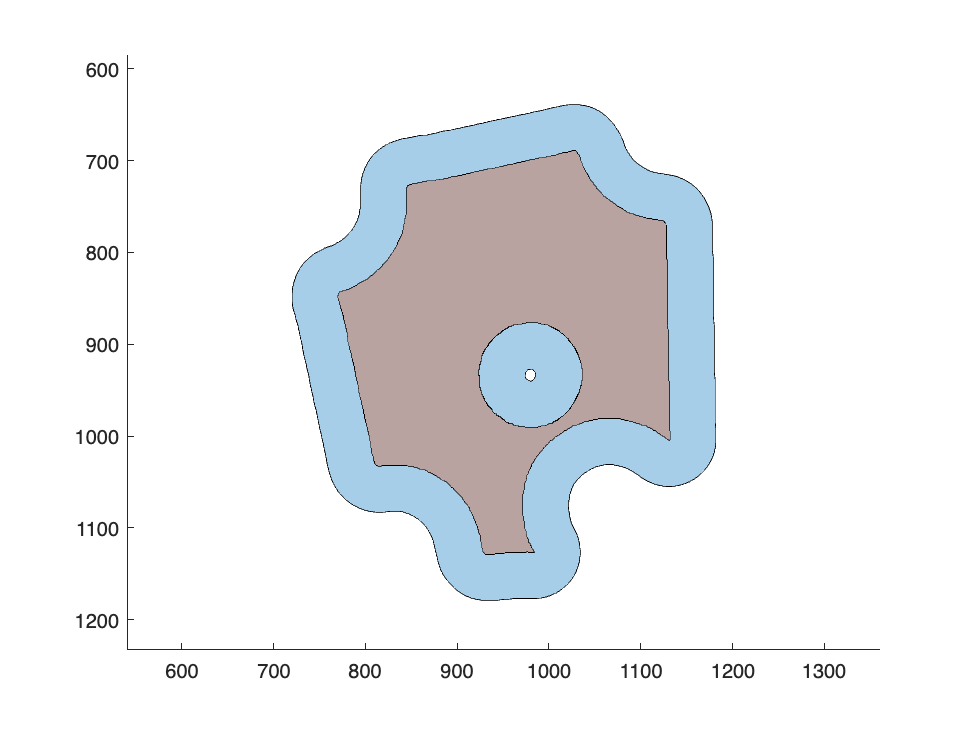# Puzzle Pieces and Polyshapes

color segmentation, opening by reconstruction, boundary tracing, polyshapes
Earlier this summer, Cleve sent me this picture of a puzzle.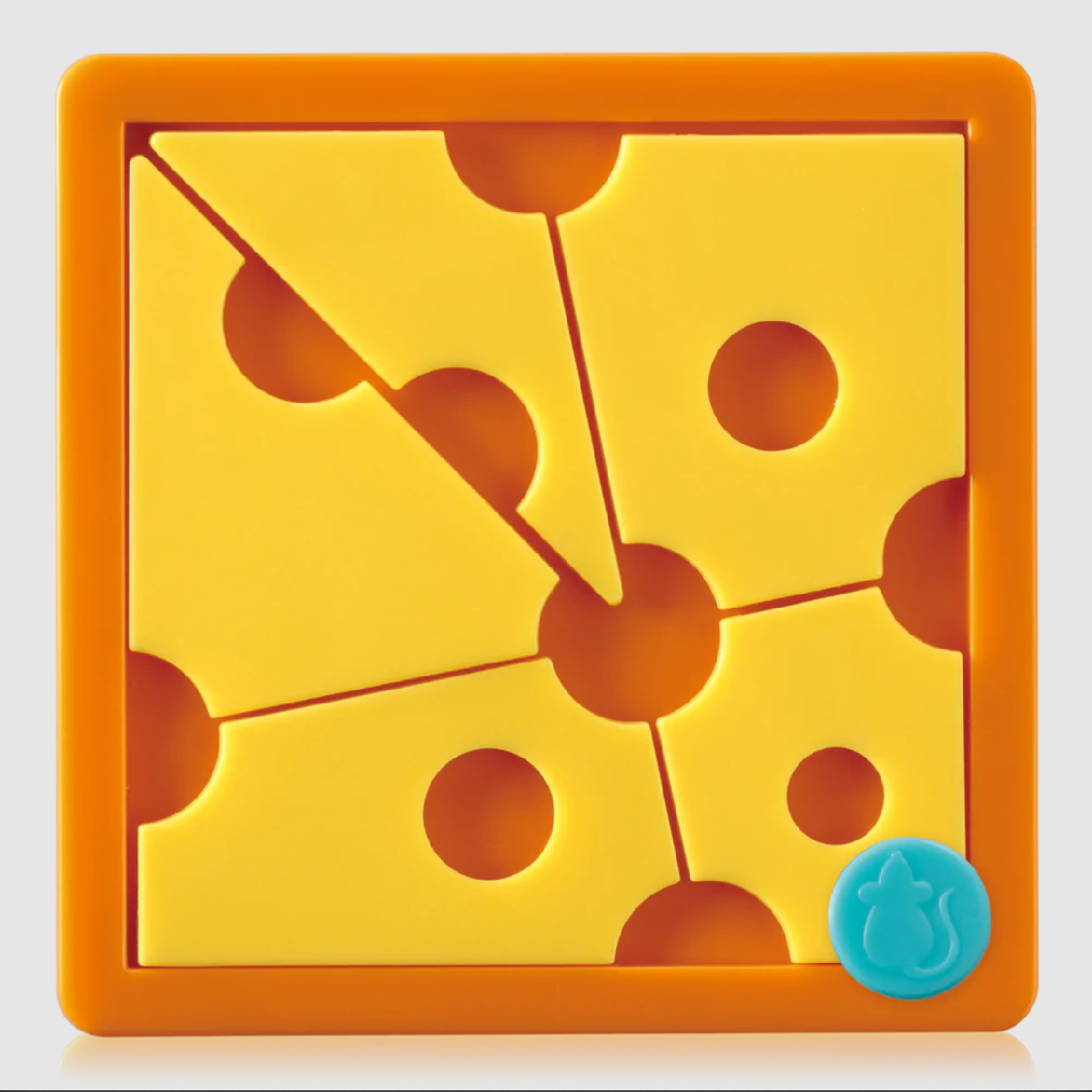He asked for tips on turning the individual "cheese slices" into patch objects for plotting and manipulation, perhaps something like this: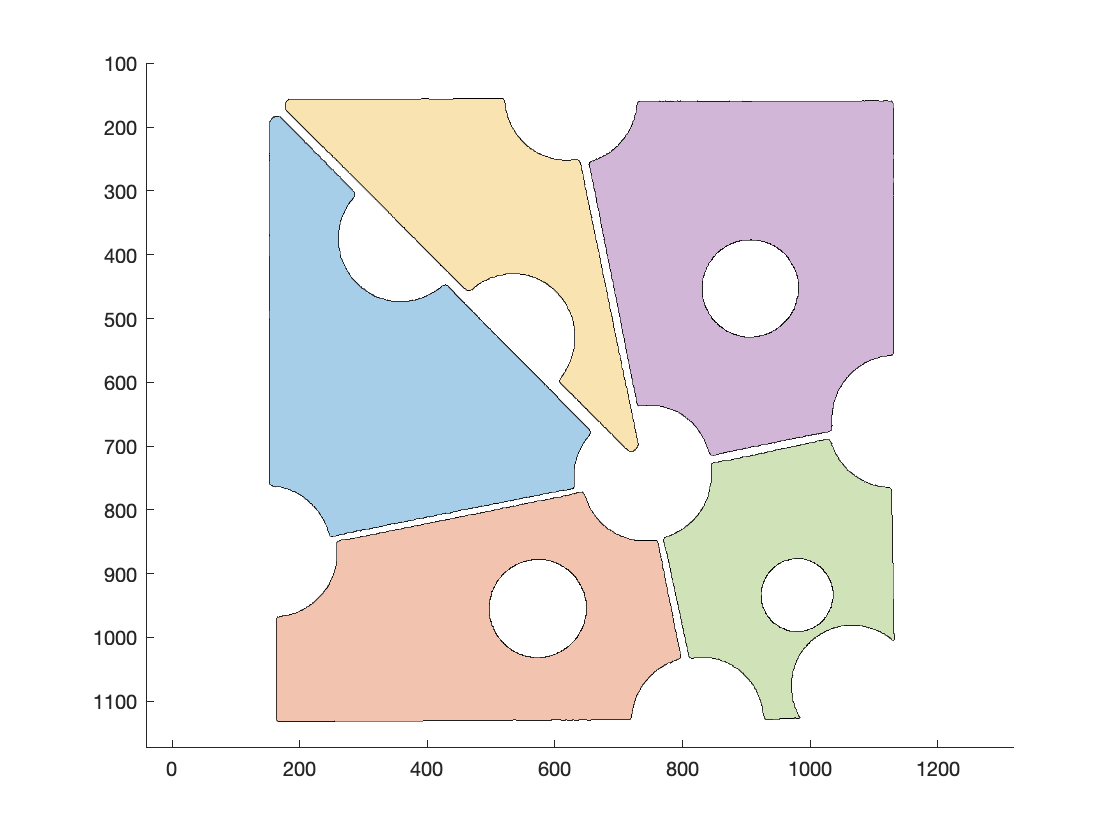In my post today, I'll elaborate on this step.
The first thing that occurred to me was to use color to segment the puzzle pieces from the rest of the image. I used the Color Thresholder app to determine some threshold values in CIELAB space.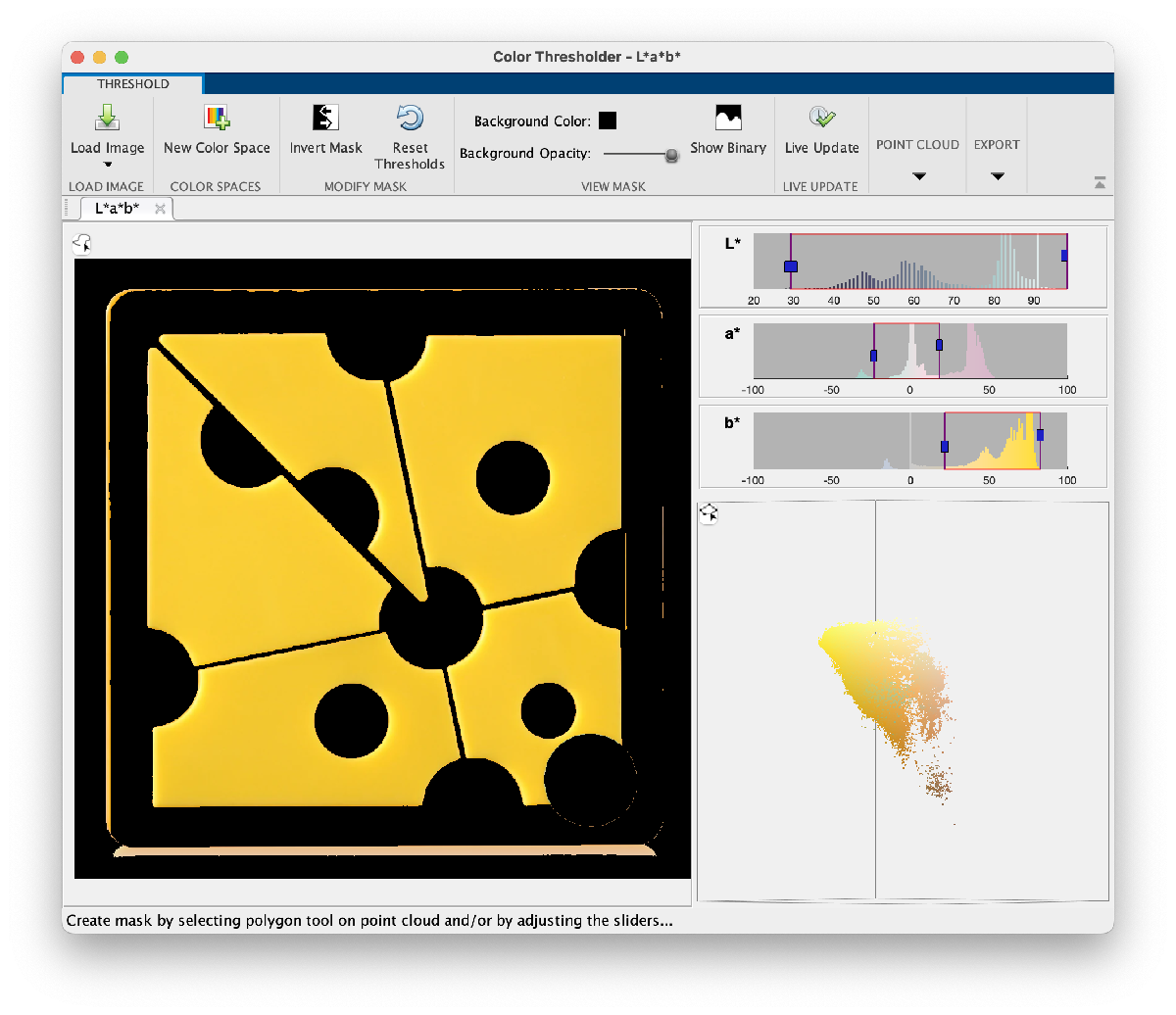lab = rgb2lab(rgb);
% Threshold values chosen with the help of colorThresholder.
[L,a,b] = imsplit(lab);
mask = ((30 <= L) & (L <= 98)) & ...
((-20 <= a) & (a <= 16)) & ...
((26 <= b) & (b <= 83));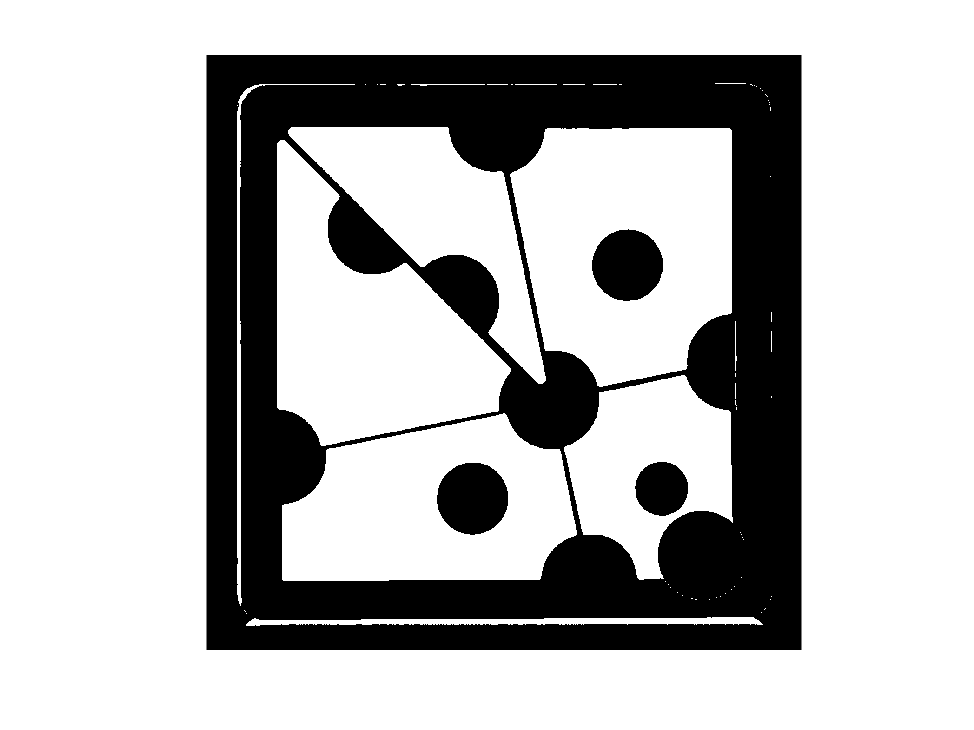Next, we need to get rid of the extra unwanted foreground pixels. I'll use a method that the mathematical morphology folks call opening by reconstruction. The first step is to erode the image in a way that eliminates all the unwanted pixels, while maintaining at least a portion of all the objects we want to keep. An erosion by a vertical line will get rid of the thin horizontal lines, and an erosion by a horizontal line will get rid of the thin vertical lines.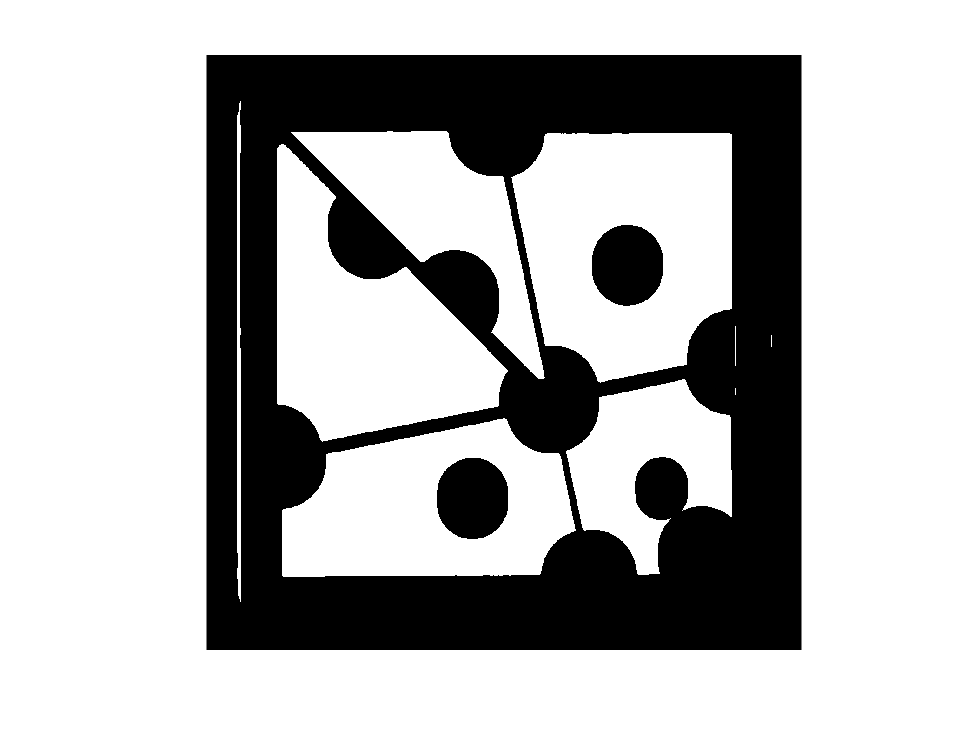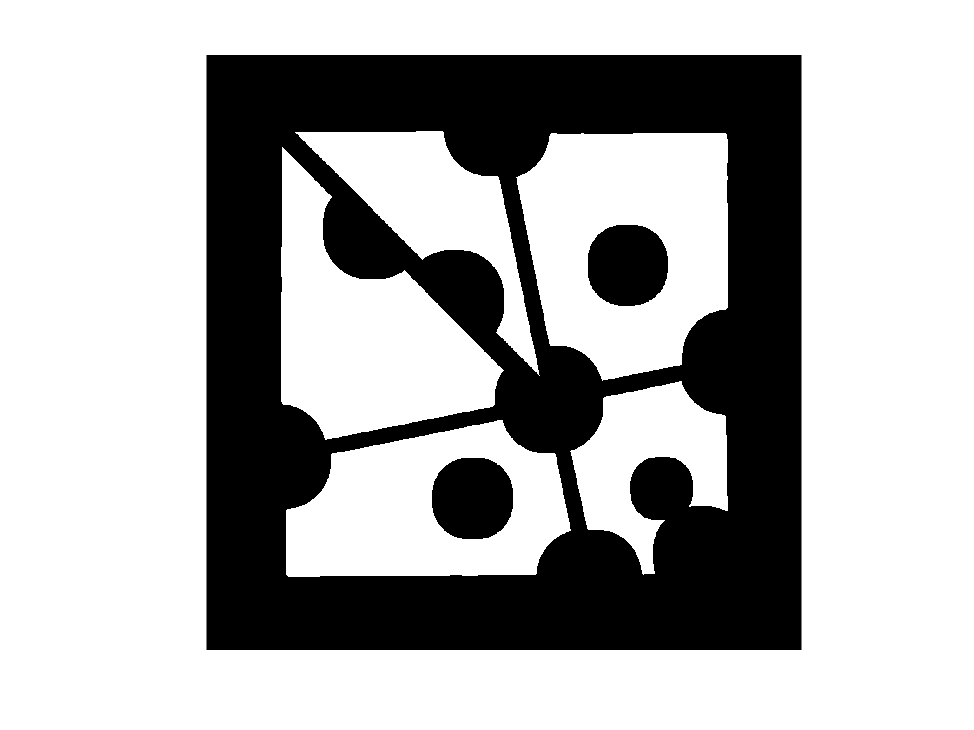In the image above, the extraneous foreground pixels are gone, but the puzzle pieces have been shrunk. I'll use morphological reconstruction to recover the entire puzzle pieces.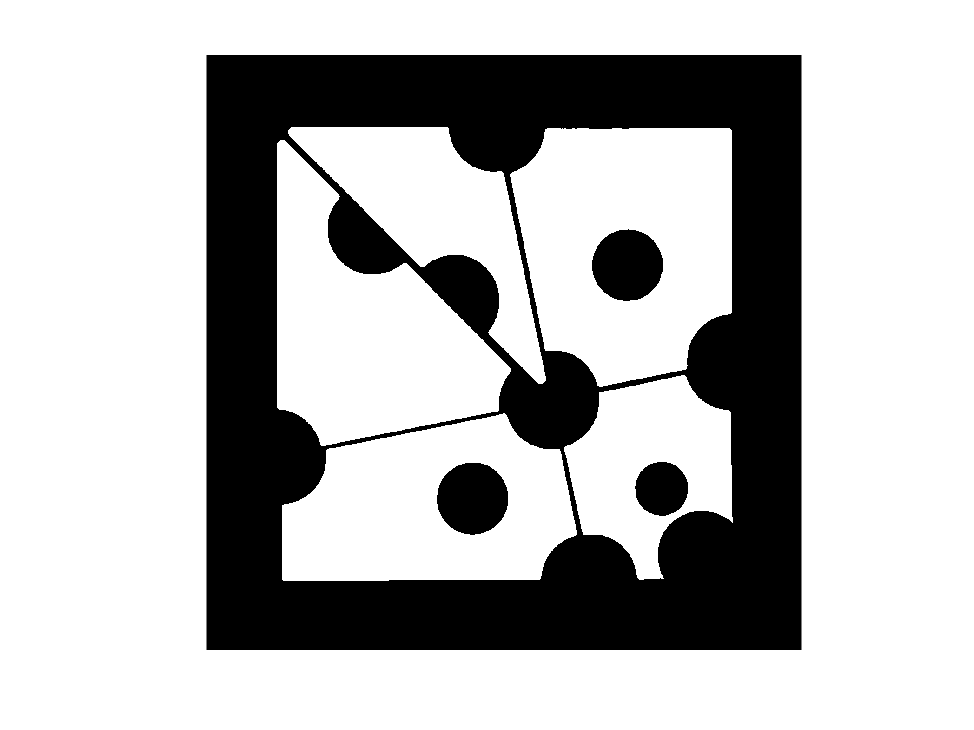Great. Now, how do we turn these puzzle pieces into some kind of easily plottable representations? Cleve had suggested patch objects, but it is complicated to create patch objects containing holes. I suggested using polyshape instead. A polyshape is an object that can represent shapes that are composed of polygons. You can create a polyshape from a collection of polygons that bound individual regions and holes, and the Image Processing Toolbox function bwboundaries can produce just such a list of polygons.
b = 9×1 cell
1
11820×2 double
21687×2 double
31669×2 double
41771×2 double
51328×2 double
6613×2 double
7609×2 double
8457×2 double
9[472,980;472,980]
The polyshape function can take a collection of bounding polygons and automatically figure out which polygons bound regions and which bound holes, but the form of the polyshape input arguments is a bit different from what bwboundaries produces. Here is some code to convert the bwboundaries output into something that polyshape can handle.
for k = 1:length(b)
X{k} = b{k}(:,2);
Y{k} = b{k}(:,1);
end
ps = polyshape(X,Y)
Warning: Boundaries with less than 3 points were removed.
Warning: Polyshape has duplicate vertices, intersections, or other inconsistencies that may produce inaccurate or unexpected results. Input data has been modified to create a well-defined polyshape.
ps =
polyshape with properties: Vertices: [3529×2 double] NumRegions: 6 NumHoles: 3
Well, that's a bit messy. In my image processing work, I often see this warning message from polyshape. Usually it is because I'm passing a bunch of colinear vertices to polyshape. They are colinear because the vertices are on the image pixel grid. I generally ignore the warning.
There's another issue, though. Why does the output of polyshape say it has 6 regions instead of 5? I had to search a bit for the reason. We can see it by zooming into one of the corners of the lower right puzzle piece and superimposing the boundary traced by bwboundaries.
figure
hold on
plot(b{5}(:,2),b{5}(:,1),'b')
hold off
xlim([1120 1140])
ylim([990 1010])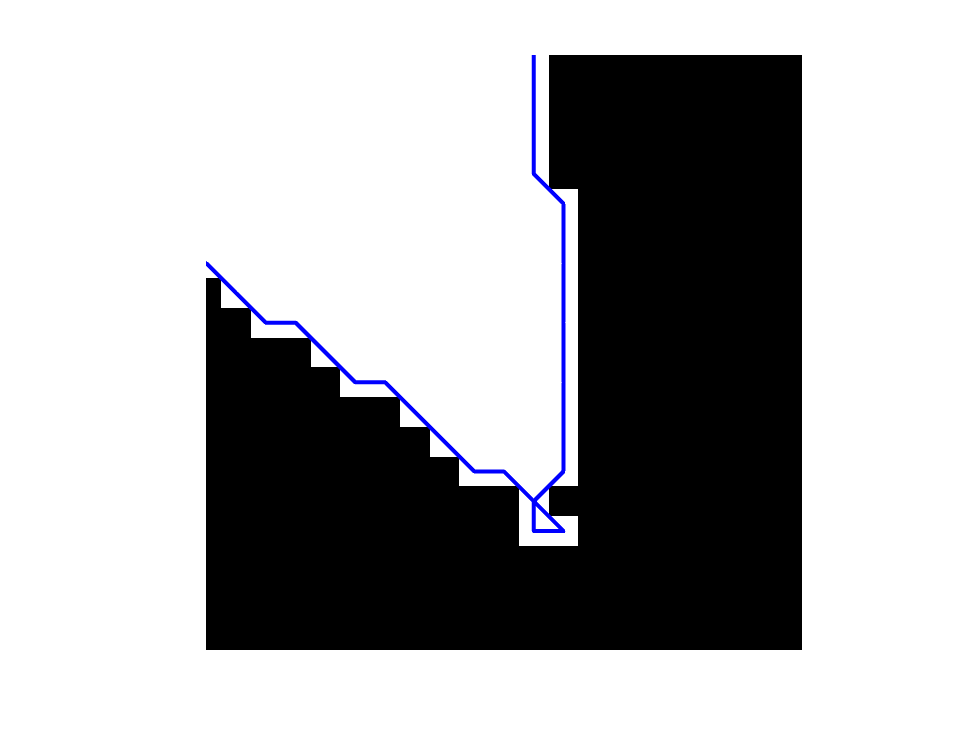There's a spot where the traced boundary is self-intersecting, and that causes polyshape to treat that tiny triangle at the bottom as a separate region. We can do a little area-based processing of the polyshape to get rid of that triangle.
Split the polyshape into separate regions:
ps_regions = regions(ps)
ps_regions =
6×1 polyshape array with properties: Vertices NumRegions NumHoles
Find the area of each region:
region_areas = area(ps_regions)
region_areas = 6×1
105 ×
1.7261 1.5884 1.0799 1.9781 0.9128 0.0000
And get rid of the tiny region:
ps_regions(region_areas < 1) = []
ps_regions =
5×1 polyshape array with properties: Vertices NumRegions NumHoles
Now we can plot our puzzle pieces. If you pass an array of polyshapes to plot, it will color each one separately.
plot(ps_regions)
axis ij
axis equal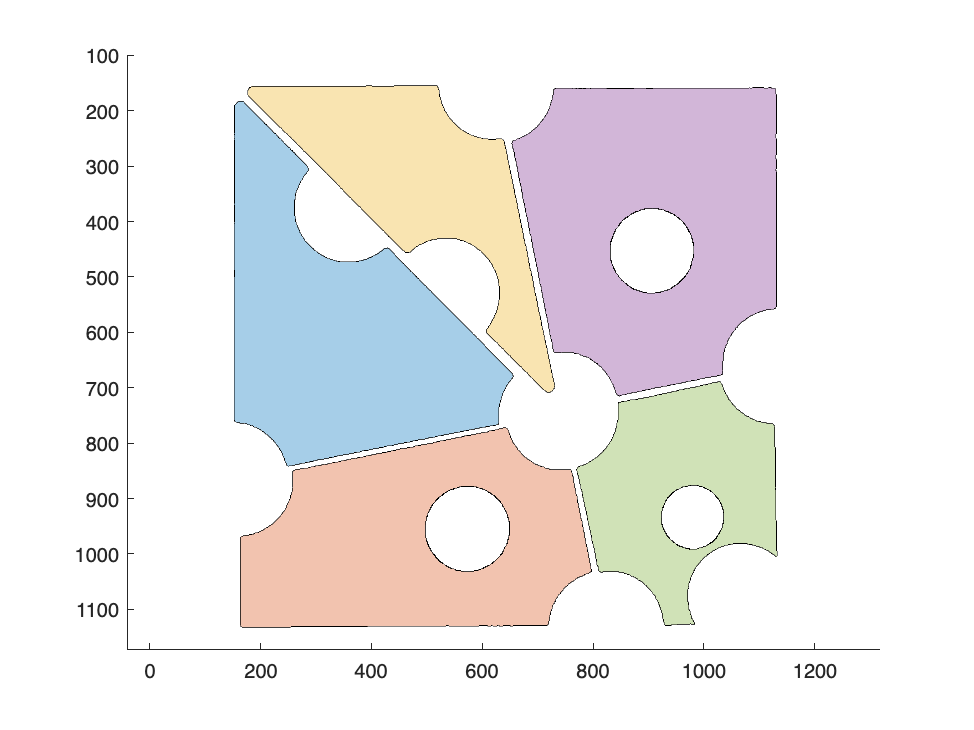I love polyshapes. They come with a rich collection of useful functions (see "Object Functions" on the polyshape reference page.) You can modify them, take them apart, join them using Boolean operations, measure them, query point locations, etc.
For example, the polybuffer function can expand (or shrink) a polyshape based on the idea of creating a "buffer zone" around the shape. To illustrate, let's take one of the puzzle pieces and give it a 50-pixel buffer zone.
ps5 = ps_regions(5);
ps5_50 = polybuffer(ps5,50);
figure
plot(ps5_50)
axis ij
axis equal
hold on
plot(ps5)
hold off